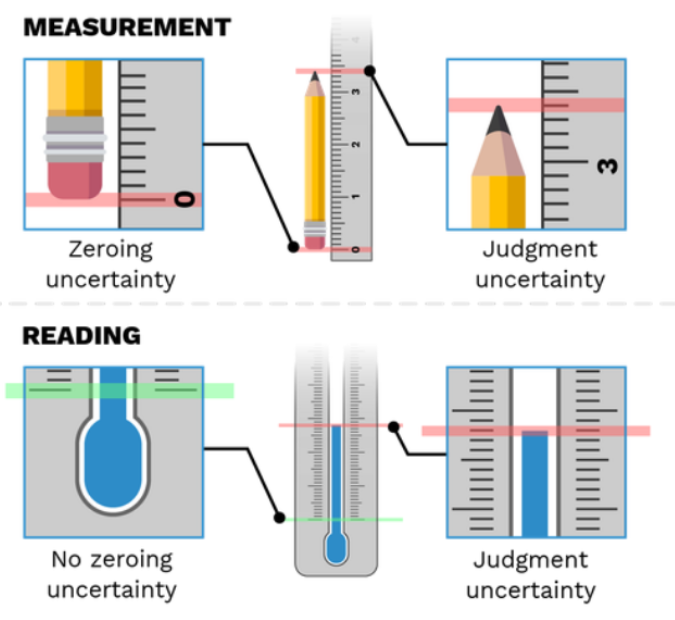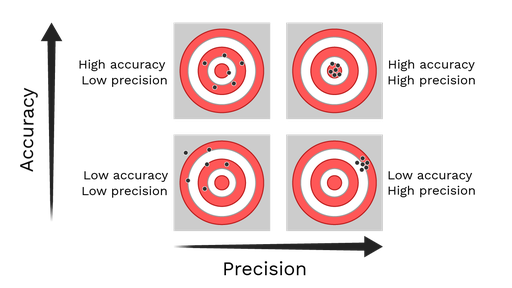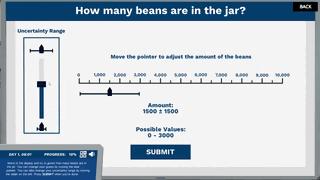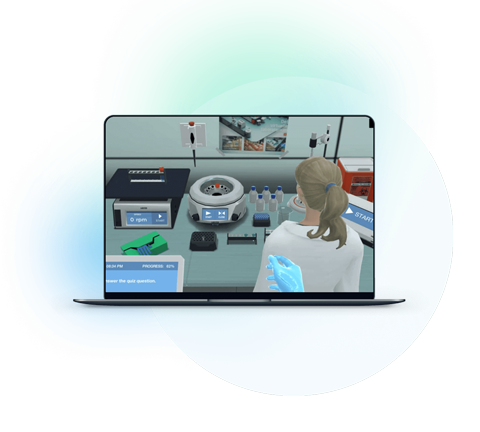Blog

# 5 Ways to Make Measurements and Uncertainty Approachable for Students

Teaching with Labster
October 11, 2022

Measurements and uncertainty are foundational concepts for all scientists, but particularly physicists. We gather information, plot the results, and attempt to draw inferences from them as we endeavor to make sense of the Universe. But to what extent can we believe them?

How can we ensure that we can have the greatest amount of faith in them? For scientists and professionals everywhere, these questions are crucial today more than ever.

When scientists explain their conclusions, they cite the data and include a measure of uncertainty to convey their confidence level. The level of uncertainty in the data tells us how correct the data is.

Every data value we obtain from certain instruments via experiments has some level of uncertainty in it. The uncertainty may be due to the assumptions we took for simplifying the problem. For instance, in most cases, we assume that Earth is a sphere but in reality, it isn’t. The uncertainty in the data may be due to personal errors when taking readings or due to any systematic error in the measuring instrument.

Whatever the reason may be, mentioning uncertainty with the results is extremely important; and so is its learning. Since it is an abstract and mathematical concept, students may find it hard to comprehend.

Read on to learn why the concept of measurement and uncertainty can be tricky for students and how you can teach it interestingly.

## Why is the concept of measurements and uncertainty tricky for Students?

Physics is an interesting subject where students explore some real-life applications. However, some abstract concepts like measurements and uncertainty can be quite tough for students. Here’s a list of three reasons why that is so:

### 1. Students find no Physical Relevance

The concept of uncertainty seems abstract to students since they can’t relate it to the real world. Moreover, they fail to understand why calculating the uncertainty of physical measurements is important for physicists. They think of it as a formality you have to follow when stating readings.

### 2. Terms like Precision and Accuracy Sound Confusing

Another hurdle for students is the technical terms. Some of the terms we use in daily life as synonyms don’t mean the same in Physics. For instance, the terms precision and accuracy are two different terms. So, it is hard for students to distinguish between them.

### 3. Combining Uncertainties can be Tricky

Calculating uncertainty is simple for most students. However, when we add, subtract, multiply or divide measurements, different rules are used to combine the uncertainties. Similarly, the more operations are involved in the calculations, the trickier it becomes to analyze and combine all the uncertainties.

## 5 Ways to Make to Get Students Energized about Measurements and Uncertainty

Keeping in view some of the hurdles faced by students, here are five ways to make measurements and uncertainty an interesting topic.

### 1. State the Importance of Uncertainty in the Real World

Students don’t know why we write an uncertainty in front of our readings or measurements. They think of it as a formality we follow when expressing measurements or readings. However, as an educator, it’s your job to state the importance of uncertainty.

Shows the Accuracy and Correctness of Data

The readings from our lab experiment won’t affect the world. However, when scientists perform experiments, their data does matter. To check the correctness of data, we check its uncertainty. The less uncertainty is, the more correct the data is and the more useful it is.

Critical Designs are Based on Measurement Uncertainty

The companies decide and plan their future tasks based on measurement uncertainty. If any of the measurements are inaccurate, or uncertainties in the values are not accommodated properly, the entire future of the organization is at risk.

Extremely Useful in Design Calculations

When designing critical parts, measurement uncertainty is quite important. If any parts don’t lie in the defined range, the whole design fails.

### 2. Explain the difference between Critical Terms with Examples

When teaching measurement and uncertainty, it is important to clarify the difference between two apparently similar terms like measurement & reading, precision & accuracy, and absolute & percentage uncertainty.

Like most terms, students use measurement and readings as synonyms. However, technically there’s a difference.

For instance, when we take a ruler and use it to determine the length of a pencil. First, we align the ruler’s zero with the pencil’s bottom and then read off the value where the pencil’s tip is. In this case, two uncertainties are involved, one is the zeroing uncertainty, and the second one is a judgment uncertainty. Such values derived from the difference between two judgments are called measurements. The values obtained using Vernier calipers, micrometer screw gauge, protractor, and ruler are measurements.

In the other case, when the instrument has a fixed zero position, and the value involves just one judgmental uncertainty. Such values are called readings. The data values obtained from thermometers, digital voltmeters, pressure gauges, and measuring cylinders, are readings.Figure from Labster Measurements & Uncertainty Simulation Showing the Difference Reading and Measurement

Precision and Accuracy

The two terms precision and accuracy are used interchangeably in English. However, in Physics, they mean something different. But students usually mix up their meanings. So, it is important to explicitly mention the difference.

Accuracy means how close a value is to the standard result. Whereas precision means how close the results are with respect to each other.

The precision and accuracy of the measurement are independent of each other. A precise measurement is not always accurate and vice versa.

A set of data that has the least uncertainty when it is both precise and accurate.Figure from Labster Showing Difference between Precision and Accuracy

The above illustration can be quite helpful when explaining precision and accuracy. This visual closely resembles a dart game. Consider the central red spot as the standard value.

In the top left image, all points are close to the red spot but they are not close to each other. That shows high accuracy but low precision.

Similarly, in the top right image, the black spots coincide with the red spot and are also close to each other. Both these conditions show high accuracy and high precision.  The same is the case with the other two images.

Absolute Uncertainty and Percentage Uncertainty

The uncertainty of a quantity mentioned in terms of its units is called absolute uncertainty. For instance, a reading from the meter rule can be 5 ± 0.1 cm. Here, 0.1 cm is the absolute uncertainty.

If we divide the absolute uncertainty by the actual measurement and multiply it by 100, we get percentage uncertainty. For instance, you can determine the percentage uncertainty in this way:

Percentage Uncertainty=0.015×100=0.2 %

### 3. Explain How to Determine the Uncertainties

Telling students how to determine the uncertainties of a different measuring instrument is necessary. To explain this, you can divide the data set from instruments into two categories; measurements and readings.

Uncertainty in a Measurement (Scaled Device)

The uncertainty in measurement from a scaled device like a ruler, screw gauge, micrometer, etc. is equal to its resolution/least count. (The resolution is the minimum value that can be measured from that particular instrument).

For instance, the Vernier caliper’s resolution is 0.01 cm; the uncertainty in its measured value is plus-minus 0.01 cm.

Uncertainty in a Reading (Digital Device)

The uncertainty in the reading from a digital device, such as a digital voltmeter, is half its resolution.  For instance, if the resolution of a device is 0.1 V, then the uncertainty in its reading would be plus-minus 0.05 V.

### 4. Mention Some Ways to Combine Uncertainties

Calculating uncertainties of individual data values is simple and depends on the least count of the measuring instrument. However, the problem arises, when we have data values with different uncertainties and we want to add, subtract, multiply or divide them. Then, what happens to the uncertainty of the final result? That’s where students are often confused.

Here are a few rules you can mention to students:

1. Absolutes Uncertainties are added when measurements are either added or subtracted.

2. When data values are divided or subtracted, their percentage uncertainties are added.

3. If a measurement is raised to some power, then to obtain uncertainty in the final result, the power/exponent is multiplied by the percentage uncertainty

4. When the average of data values is taken, the final uncertainty is also the average of all absolute uncertainties.

### 5. Use Virtual Lab Simulations

Experiments in the lab are the best way to understand Physics concepts. One way is to design an experiment where students measure some lengths with different devices and then calculate the absolute and percentage uncertainties in their readings.

However, the same experiments can be done through simulations.  Labster provides you with exciting virtual simulations that engage students in topics through learning scenarios. We teach theoretical concepts through visual experiences.In this case, you can use Labster Measurements and Uncertainty Simulation, in which students learn the selection of the right tools for their experiment and how they can reduce uncertainty in their results.